User:Tohline/PGE/RotatingFrame

NOTE to Eric Hirschmann & David Neilsen... I have move the earlier contents of this page to a new Wiki location called Compressible Riemann Ellipsoids.

Rotating Reference Frame

 |   Tiled Menu   |   Tables of Content   |  Banner Video   |  Tohline Home Page   |

At times, it can be useful to view the motion of a fluid from a frame of reference that is rotating with a uniform (i.e., time-independent) angular velocity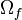$~\Omega_f$. In order to transform any one of the principal governing equations from the inertial reference frame to such a rotating reference frame, we must specify the orientation as well as the magnitude of the angular velocity vector about which the frame is spinning,${\vec\Omega}_f$; and the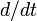$~d/dt$ operator, which denotes Lagrangian time-differentiation in the inertial frame, must everywhere be replaced as follows: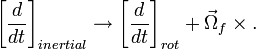$\biggl[\frac{d}{dt} \biggr]_{inertial} \rightarrow \biggl[\frac{d}{dt} \biggr]_{rot} + {\vec{\Omega}}_f \times .$

Performing this transformation implies, for example, that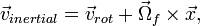$\vec{v}_{inertial} = \vec{v}_{rot} + {\vec{\Omega}}_f \times \vec{x} ,$

and,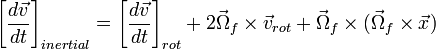$\biggl[ \frac{d\vec{v}}{dt}\biggr]_{inertial} = \biggl[ \frac{d\vec{v}}{dt}\biggr]_{rot} + 2{\vec{\Omega}}_f \times {\vec{v}}_{rot} + {\vec{\Omega}}_f \times ({\vec{\Omega}}_f \times \vec{x})$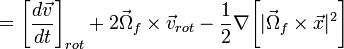$= \biggl[ \frac{d\vec{v}}{dt}\biggr]_{rot} + 2{\vec{\Omega}}_f \times {\vec{v}}_{rot} - \frac{1}{2} \nabla \biggl[ |{\vec{\Omega}}_f \times \vec{x}|^2 \biggr]$

(If we were to allow${\vec\Omega}_f$ to be a function of time, an additional term involving the time-derivative of${\vec\Omega}_f$ also would appear on the right-hand-side of these last expressions; see, for example, Eq.~1D-42 in BT87.) Note as well that the relationship between the fluid vorticity in the two frames is,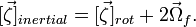$[\vec\zeta]_{inertial} = [\vec\zeta]_{rot} + 2{\vec\Omega}_f .$

Continuity Equation (rotating frame)

Applying these transformations to the standard, inertial-frame representations of the continuity equation presented elsewhere, we obtain the:

Lagrangian Representation
of the Continuity Equation
as viewed from a Rotating Reference Frame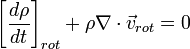$\biggl[ \frac{d\rho}{dt} \biggr]_{rot} + \rho \nabla \cdot {\vec{v}}_{rot} = 0$ ;

Eulerian Representation
of the Continuity Equation
as viewed from a Rotating Reference Frame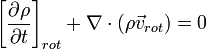$\biggl[ \frac{\partial\rho}{\partial t} \biggr]_{rot} + \nabla \cdot (\rho {\vec{v}}_{rot}) = 0$ .

Euler Equation (rotating frame)

Applying these transformations to the standard, inertial-frame representations of the Euler equation presented elsewhere, we obtain the:

Lagrangian Representation
of the Euler Equation
as viewed from a Rotating Reference Frame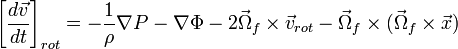$\biggl[ \frac{d\vec{v}}{dt}\biggr]_{rot} = - \frac{1}{\rho} \nabla P - \nabla \Phi - 2{\vec{\Omega}}_f \times {\vec{v}}_{rot} - {\vec{\Omega}}_f \times ({\vec{\Omega}}_f \times \vec{x})$ ;

Eulerian Representation
of the Euler Equation
as viewed from a Rotating Reference Frame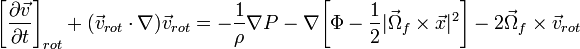$\biggl[\frac{\partial\vec{v}}{\partial t}\biggr]_{rot} + ({\vec{v}}_{rot}\cdot \nabla) {\vec{v}}_{rot}= - \frac{1}{\rho} \nabla P - \nabla \biggl[\Phi - \frac{1}{2}|{\vec{\Omega}}_f \times \vec{x}|^2 \biggr] - 2{\vec{\Omega}}_f \times {\vec{v}}_{rot}$ ;

Euler Equation
written in terms of the Vorticity and
as viewed from a Rotating Reference Frame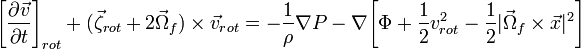$\biggl[\frac{\partial\vec{v}}{\partial t}\biggr]_{rot} + ({\vec{\zeta}}_{rot}+2{\vec\Omega}_f) \times {\vec{v}}_{rot}= - \frac{1}{\rho} \nabla P - \nabla \biggl[\Phi + \frac{1}{2}v_{rot}^2 - \frac{1}{2}|{\vec{\Omega}}_f \times \vec{x}|^2 \biggr]$ .

Centrifugal and Coriolis Accelerations

Following along the lines of the discussion presented in Appendix 1.D, §3 of BT87, in a rotating reference frame the Lagrangian representation of the Euler equation may be written in the form,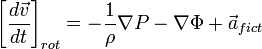$\biggl[ \frac{d\vec{v}}{dt}\biggr]_{rot} = - \frac{1}{\rho} \nabla P - \nabla \Phi + {\vec{a}}_{fict}$,

where,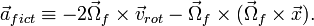${\vec{a}}_{fict} \equiv - 2{\vec{\Omega}}_f \times {\vec{v}}_{rot} - {\vec{\Omega}}_f \times ({\vec{\Omega}}_f \times \vec{x}) .$

So, as viewed from a rotating frame of reference, material moves as if it were subject to two fictitious accelerations which traditionally are referred to as the,

Coriolis Acceleration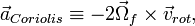${\vec{a}}_{Coriolis} \equiv - 2{\vec{\Omega}}_f \times {\vec{v}}_{rot} ,$

(see the related Wikipedia discussion) and the

Centrifugal Acceleration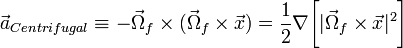${\vec{a}}_{Centrifugal} \equiv - {\vec{\Omega}}_f \times ({\vec{\Omega}}_f \times \vec{x}) = \frac{1}{2} \nabla\biggl[ |{\vec{\Omega}}_f \times \vec{x}|^2 \biggr]$

(see the related Wikipedia discussion).

Nonlinear Velocity Cross-Product

In some contexts — for example, our discussion of Riemann ellipsoids or the analysis by Korycansky & Papaloizou (1996) of nonaxisymmetric disk structures — it proves useful to isolate and analyze the term in the "vorticity formulation" of the Euler equation that involves a nonlinear cross-product of the rotating-frame velocity vector, namely,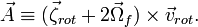$\vec{A} \equiv ({\vec{\zeta}}_{rot}+2{\vec\Omega}_f) \times {\vec{v}}_{rot} .$

NOTE: To simplify notation, for most of the remainder of this subsection we will drop the subscript "rot" on both the velocity and vorticity vectors.

Align${\vec\Omega}_f$ with z-axis

Without loss of generality we can set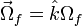${\vec\Omega}_f = \hat{k}\Omega_f$, that is, we can align the frame rotation axis with the z-axis of a Cartesian coordinate system. The Cartesian components of${\vec{A}}$ are then,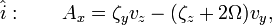$\hat{i}: ~~~~~~ A_x = \zeta_y v_z - (\zeta_z + 2\Omega) v_y ,$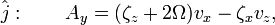$\hat{j}: ~~~~~~ A_y = (\zeta_z + 2\Omega) v_x - \zeta_x v_z ,$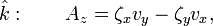$\hat{k}: ~~~~~~ A_z = \zeta_x v_y - \zeta_y v_x ,$

where it is understood that the three Cartesian components of the vorticity vector are,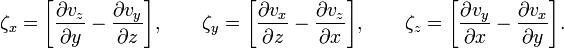$\zeta_x = \biggl[\frac{\partial v_z}{\partial y} - \frac{\partial v_y}{\partial z} \biggr] , ~~~~~~ \zeta_y = \biggl[ \frac{\partial v_x}{\partial z} - \frac{\partial v_z}{\partial x} \biggr] , ~~~~~~ \zeta_z = \biggl[ \frac{\partial v_y}{\partial x} - \frac{\partial v_x}{\partial y} \biggr] .$

In turn, the curl of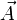$\vec{A}$ has the following three Cartesian components: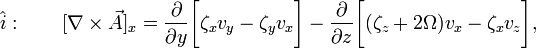$\hat{i}: ~~~~~~ [\nabla\times\vec{A}]_x = \frac{\partial}{\partial y}\biggl[ \zeta_x v_y - \zeta_y v_x \biggr] - \frac{\partial}{\partial z}\biggl[ (\zeta_z + 2\Omega) v_x - \zeta_x v_z \biggr],$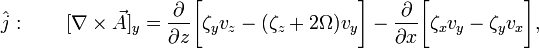$\hat{j}: ~~~~~~ [\nabla\times\vec{A}]_y = \frac{\partial}{\partial z}\biggl[ \zeta_y v_z - (\zeta_z + 2\Omega) v_y \biggr] - \frac{\partial}{\partial x}\biggl[ \zeta_x v_y - \zeta_y v_x \biggr] ,$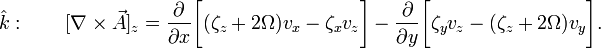$\hat{k}: ~~~~~~ [\nabla\times\vec{A}]_z = \frac{\partial}{\partial x}\biggl[ (\zeta_z + 2\Omega) v_x - \zeta_x v_z \biggr] - \frac{\partial}{\partial y}\biggl[ \zeta_y v_z - (\zeta_z + 2\Omega) v_y \biggr] .$

When vz = 0

If we restrict our discussion to configurations that exhibit only planar flows — that is, systems in which vz = 0 — then the Cartesian components of${\vec{A}}$ and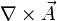$\nabla\times\vec{A}$ simplify somewhat to give, respectively,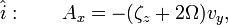$\hat{i}: ~~~~~~ A_x = - (\zeta_z + 2\Omega) v_y ,$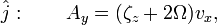$\hat{j}: ~~~~~~ A_y = (\zeta_z + 2\Omega) v_x ,$$\hat{k}: ~~~~~~ A_z = \zeta_x v_y - \zeta_y v_x ,$

and,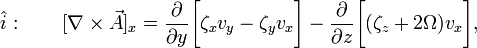$\hat{i}: ~~~~~~ [\nabla\times\vec{A}]_x = \frac{\partial}{\partial y}\biggl[ \zeta_x v_y - \zeta_y v_x \biggr] - \frac{\partial}{\partial z}\biggl[ (\zeta_z + 2\Omega) v_x \biggr],$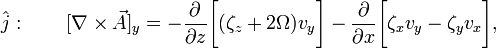$\hat{j}: ~~~~~~ [\nabla\times\vec{A}]_y = - \frac{\partial}{\partial z}\biggl[(\zeta_z + 2\Omega) v_y \biggr] - \frac{\partial}{\partial x}\biggl[ \zeta_x v_y - \zeta_y v_x \biggr] ,$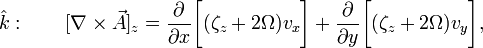$\hat{k}: ~~~~~~ [\nabla\times\vec{A}]_z = \frac{\partial}{\partial x}\biggl[ (\zeta_z + 2\Omega) v_x \biggr] + \frac{\partial}{\partial y}\biggl[ (\zeta_z + 2\Omega) v_y \biggr] ,$

where, in this case, the three Cartesian components of the vorticity vector are,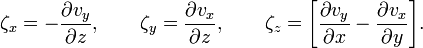$\zeta_x = - \frac{\partial v_y}{\partial z} , ~~~~~~ \zeta_y = \frac{\partial v_x}{\partial z} , ~~~~~~ \zeta_z = \biggl[ \frac{\partial v_y}{\partial x} - \frac{\partial v_x}{\partial y} \biggr] .$

Related Discussions

 © 2014 - 2019 by Joel E. Tohline |   H_Book Home   |   YouTube   | Context: | PGE | SR | Appendices: | Equations | Variables | References | Binary Polytropes | Ramblings | Images | Images (2016 Layout) | ADS |